Parası kalmadığı için otobüse binemiyordur ailesi porno izle ona daha yeni para gönderdiği için tekrar porno istemeye utanınca mecburen otostop çekmek için youporn çantasını alarak yol kenarına gelir etekli porno liseli türk kız yol kenarında dururken yanına yaklaşan porno kibar bir gencin onu gideceği yere kadar bırakmak porno izle istemesine çok mutlu olur arabaya bindiklerinde gideceği yer ile porno arabayı kullanan adamın gittiği yer arasında çok mesafe sex izle farkı olduğunu anlayan türk kız bu yaptığı porno indir iyilik karşısında arabada ona memelerini açar porno sapıklaşan adam yol kenarındaki hotelde durarak porno izle üniversiteli otostop çeken türk kızına odada sakso çektirip sikerTotal Pathos Edge Semi Entire Block Graph
All submissions of the EM system will be redirected to Online Manuscript Submission System. Authors are requested to submit articles directly to Online Manuscript Submission System of respective journal.

Original Article
, Volume: 15( 3)

# Total Pathos Edge Semi Entire Block Graph

*Correspondence:
Venkanagouda M Goudar , Department of Mathematics, Sri Siddhartha Institute of Technology, Tumkur, Karnataka, India, Tel: 9448579479; E-mail: [email protected]

Received: May 12, 2017; Accepted: June 21, 2017; Published: June 26, 2017

Citation: Venkanagouda M Goudar and Jagadeesh N. Total Pathos Edge Semi Entire Block Graph. Int J Chem Sci. 2017;15(3):151.

### Abstract

In this paper, we introduce the concept of total pathos edge semi entire block graph of a tree. We obtain some properties of this graph. We study the characterization of graphs whose total pathos edge semi entire block graphs are always nonplanar, crossing number one, Eulerian and Hamiltonian.

### Keywords

Block graph; Edge Semientire graph; Inner vertex number; Line graph

### Introduction

Let G (p,q) be a connected graph with vertex set V=p and the edge set E=q. If e=uv is in E (G), then the vertices u and v are adjacent. For the graph theoretical notation, we follow in [1,2].

In  Venkanagouda et al. introduced the graph valued function, the pathos edge semi entire block graph of a tree T. The block graph B (G) of a graph G was introduced in . Further the path graph P (T) of a tree was studied in .

The following theorems will be used in the sequel.

Theorem 1 . If G is a (p, q) graph whose vertices have degree di then L (G) has q vertices and qL edges whereTheorem 2 . The line graph L (G) of a graph G has crossing number one if and only if G is planar and 1 or 2 holds:

1. The maximum degree d (G) is 4 and there is unique non-cut vertex of degree 4.

2. The maximum degree d (G) is 5, every vertex of degree 4 is a cut vertex and there is a unique vertex of degree 5 and has at most 3 edges in any block.

Theorem 3 . A connected graph G is isomorphic to its line graph if and only if it is a cycle .

Theorem 4 . The line graph L (G) of a graph is planar if and only if G is planar, Δ ≤ 4 and if deg v=4 for a vertex v of G, then v is a cut vertex.

Theorem 5 . A graph is planar if and only if it has no subgraph homeomorphic to K5 or K3,3.

Theorem 6 . For any planar graph G, edge semi entire block graph Eb (G) whose vertices have degree di has (q+r+b) vertices and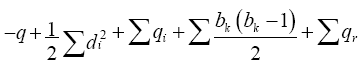edges, where r the number of regions, b be the number of blocks, qj the number of edges in a block bj, bk be the blockdegree of a cut vertex Ck and qr be the number of edges lies on the region r1.

Theorem 7 . For any planar graph G, pathos edge semi entire block graph PEb (G) whose vertices have degree di has (2q+k+1) vertices andedges, where r be the number of regions, b the number of blocks, qj the number of edges lies in a block bj, bk be the block degree of a cut vertex Ck and qr be the number of edges lies in the region r1.

Theorem 8 . A connected graph G is eulerian if and only if each vertex in G has even degree .

Theorem 9 . A nontrivial graph is bipartite if and only if all its cycles are even.

Theorem 10 . For any tree T, the pathos edge semi entire block graph PEb (T) is planar if and only if T is a star graph K1, n, for n ≥ 3.

### Total Pathos Edge Semi Entire Block Graph

In this section, we define the total pathos edge semi entire block graph of a tree.

Definition 2.1 The total pathos edge semi entire block graph of a tree T denoted by TPe (T) is the graph whose vertex set is the collection of edges, blocks, regions and path of pathos of T in which two vertices of TPe (T) are adjacent if both are the edges of T which are adjacent or both are blocks of T which are adjacent or one is a block and other is an edge e of T and e lies on it, or one is a region and other is an edge e of T and edge e lies on the region or one is a path of pathos of T and other is an edge e of T in which e lies on the path or both are the path of paths pi and pj of T and both pi and pj have a common cut vertex. In Figure 1, a graph G and its total pathos edge semi entire block graph are shown .

We begin with the following direct results.

Remark 1. For any tree T,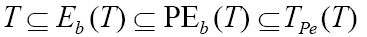Remark 2. For any graph G, Tpe (G)=PEb (T)U P (T)

Remark 3. For any edge ei in T with edge degree n, the degree of the vertex e’i which corresponds to ei in TPe (T) is always n+1.

### Results

Theorem 11. Let T be a tree, the total pathos edge semi entire block graph TPe (T) is always non-separable.

Proof. Consider T be a tree and let ei for all i?T be the edges of T. In a Tree T, every edge becomes a block. Let b1=e1, b2=e2. bm=em be the blocks, r1 be the only one region in T and p1, p2. pk be the pathos of T. By the definition of L (T), the vertices e1, e2. em of L (T) form a sub graph without cut vertex. Also in TPe (T), the vertex formed from the region called the region vertex is adjacent to ei for all i=1,2. m, to form a nonseparable graph. Since there are m blocks which are K2, we have each bi is adjacent to ei for all i.

Further T contains some pendent pathos, these correspond to the pathos vertices pi and each pi is adjacent to only one vertex ej such that ej becomes a cut vertex. Also in a tree, at least two paths are always adjacent then TPe (T) is non-separable .

Theorem 12. If T (p, q) is a connected graph whose vertices have degree di and if the number of blocks to which the edge ei belongs in T is bi, then the total pathos edge semi entire block graph TPe (T) has 2q+k+1 vertices and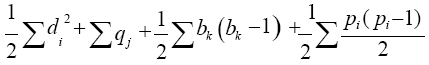edges, where qj be the number of edges in each block bjand bk be the block degree of a cut vertex ck.

Proof. By Remark 1,Thus, the number of vertices in TPe (T) equals the number of vertices of of PEb (T). By theorem 7, V[PEb (T)]=2q+k+1.

Further by Remark 2, the number of edges in TPe (T) is same as the number of edges in PEb (T) and the number of edges P(T).

By theorem 7, the number of edges in PEb (T) is. Also, the number of edges in a path graph P(T) is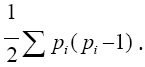Hence the number of edgesTheorem 13. For any tree T, TPe (T) is non-complete graph.

Proof. By definition of TPe (T), there is no adjacency between the regions and blocks. In TPe (T) there is no edge between the region vertex and block vertex. Hence TPe (T) is non-complete graph.

Theorem 14. For any tree T, the total pathos edge semi entire block graph TPe (T) is not a bipartite graph.

Proof. In a tree other than path contains at least one vertex v of T such that degree of v ≥ 3. Let e1, e2, e3 be the edges incident to v. By the definition of TPe (T), the edges incident with v, form a cycle C3 in TPe (T). By Theorem 9, TPe (T) is not bipartite graph.

Theorem 15. For any tree T, TPe (T) ? PEb (T) if and only if T is a path.

Proof. Let T be a path. By definition, TPe (T), PEb (T) have the same number of vertices. Since T is a path and the pathos graph of a path has no edges, it implies by definitions that TPe (T) and PEb (T) are isomorphic.

Conversely suppose TPe (T) ? PEb (T) and T is non-trivial connected tree. We now prove that T is a path. We assume that T has at least one vertex v such that degree of v ≥ 3. By remark 2, the number of edges in TPe (T) is the sum of the number of edges PEb (T) and the number of edges in P (T). Hence the number of edges in PEb (T) is less than the number of edges in TPe (T). Thus TPe (T) ? PEb (T), a contradiction. Thus, T is a path.

Theorem 16. For any tree T, total pathos edge semi entire block graph TPe (T) is always nonplanar.

Proof. Let T be a connected tree and be a star K1, n, for n ≤ 3. By Theorem 10, PEb (T) is planar. Let e1=b1, e2=b2, and e3=b3 be the edges which are also blocks, r1 be the region and p1, p2 be the path of pathos of T. In TPe (T), the vertices e1, e2, e3 and r1 form a complete graph K4. The block vertices b1, b2, b3 form a complete graph K3 and the edges between p1 and p2 must crosses the edges already drawn and hence TPe (T) is nonplanar.

If T=K1, n, n ≥ 4, by the Theorem 10, PEb (T) is nonplanar and hence TPe (T) is nonplanar.

Theorem 17. For any tree T, the total pathos edge semi entire block graph TPe (T) has crossing number one if and only if T is K1, 3.

Proof. Suppose TPe (T) has crossing number one, clearly TPe (T) is nonplanar. By Theorem 16, we have T=K1, n, n ≥ 4. Assume that T=K1, n for n=4. By the definition of L (T), L (K1,4)=K4. Since every edge of T lies on only one region r1 so in TPe (T), all vertices of K4 are adjacent to the region vertex r1 to form K5, clearly it has crossing number one. Further in a tree T, each edge is a block and all blocks b1, b2, b3 and b4 are adjacent to each other. By the definition of TPe (T), all four block vertices form K4 as sub graph, clearly the inner vertex bi is adjacent to the edge ei which corresponds to e’i in TPe (T) which form another one crossing number. Hence TPe (T) has crossing number at least two, a contradiction [9,10].

Proving conversely, show that K5 is a subgraph which has crossing number one. By the definition of line graph, L (K1,3)=K3 and all edges lies on only one region. In TPe (T), the region vertex r1 is adjacent to all vertices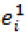which corresponds to the edges of T and it form a complete graph K4 . Further each edge is a block and all blocks form K3 as a sub graph. Also, the pathos vertices p1 adjacent is e1 and e2, p2 is adjacent to e3 and these pathos vertices are adjacent to each other and it form crossing number one. Hence, Cr [ TPe (T)]=1.

Theorem 18. For any tree T, the total pathos edge semientire block graph TPe (T) is Eulerian if and only if T is a star K1, 4n+2 for n ≥ 1.

Proof. Suppose TPe (T) is Eulerian. We consider the following cases.

Case 1. Assume that T be any non-star tree T, we have the following sub cases.

Sub case 1.1. Let u, v ?T such that degree (u)=3 and degree (v)=2. Let e1, e2, e3 be the edges incident to u and e3, e4 be the edges incident to v. Clearly edge degree of an edge e1 is 4. By the Remark 3, the corresponding vertexin TPe (T) have degree 5, which is odd. By Theorem 8, TPe (T) is noneulerian, a contradiction.

Sub case 1.2. Assume that T contain an edge have edge degree of every edge is even, which is possible only when the total number of edges of T will be odd. By definition of TPe (T), the deg (ri) becomes odd. By Theorem 8, TPe (T) is noneulerian, a contradiction.

Case 2. Assume that T=K1, p where p ≠ 4n+2. We have the following subcases.

Sub case 2.1. We assume that T=K1, 3n+1 n=1.2. k. By case 1, each edge of K1, 3 have edge degree 4, by Theorem 2, the corresponding vertices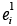in TPe (T) have edge degree odd, which is odd. By the Theorem 8, TPe (T) is noneulerian, a contradiction.

Sub case 2.2. Assume that T=K1,4. By case 1, each edge ei in T have edge degree odd. By the Remark 3, the corresponding vertices e1 in TPe (T) have even degree. The region vertex r’i is adjacent to all four vertices v1i , i=1, 2, 3, 4 which form a graph with all vertices of even degree. In T, there are two paths of pathos p1 and p2 and each pathos contains two edges. In TPe (T), both pathos vertices adjacent to form graph with both p1 and p2 have odd degree. By Theorem 8, TPe (T) is noneulerian, a contradiction.

Conversely suppose T=K1, 4p+2 for p=1,2. n. In a tree T, every edge ei have odd degree, by Remark 3, the verticescorresponds to ei of T in TPe (T) becomes even degree. Further the total number of edges of T is even, then the region vertex in TPe (T) becomes even degree. Also, each edge is a block ei =bi for all i, each block bi adjacent to remaining blocks and ei adjacent to bi to form even degree. Lastly the pathos vertices which are adjacent to even number of edges and each pathos are adjacent to remaining 2n path of pathos. Then in TPe (T), every vertex is of even degree. Hence TPe (T) is Eulerian.

Theorem 19. For any tree T, TPe (T) is Hamiltonian.

Proof. Suppose T be a tree. We consider the following cases.

Case 1. Let T be a star K1, n, n is odd with vertices u1, u2u3.... unun+k such that degree of u1=n and let b1, b2 . . .bm be the number of blocks, ri be the regions, the number of paths of pathos areand T contains only one region. By the definition of TPe (T), V[TPe (T)]={e1, e2, e3 . . . . . .en, b1, b2, . . . . . bn-1}Then there exist a cycle containing the vertices of TPe (T) as p1,p2, p3,…pk,e5,ek,bkbk-1,. . . b1,e1,e3… r.e2 p1 and it includes all the vertices of TPe (T). Hence it is a Hamiltonian cycle.

Case 2. Let T=K1,n, n>2 and is even. Then the number of path of pathos isLet V [TPe (T)]={e1,e2 . . . .en, b1,b2. . . bn-1} U {p1, p2 . . . . . .} U r1 then there exist a cycle which contains the vertices of TPe (T) as p1, p2,…ek bk, bk-1, . . . b1,e1,…r.,e2 p1 and is a Hamilton cycle. Hence TPe (T) is Hamiltonian.

Case 3. Suppose T is neither a path nor a star, then T contains at least two vertices of degree >2. Let e1, e2. . . . .en be the edges of T such that e1=b1, e2=b2. . . . . en=bn be the blocks. Then V [TPe (T)]={e1, e2 . . . en, b1, b2. . . .bn}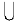{p1, p2 . . . . .pi}r1 where T has pi pathos vertices for i > 1 and each pathos vertex is adjacent to the edge of T, where the corresponding pathos lie on the edges of T. Then there exist a cycle C which contains all the vertices of TPe (T) as p1, e1b1e2p2e3. . . . . . . . . r1 en p1. Hence TPe (T) is a Hamiltonian. Clearly TPe (T) is a Hamiltonian.

Case 4. Suppose T is a path. Let u1, u2u3. . . . . un be a path. The vertex set V [TPe (T)]={e1,e2 . . . .en, b1,b2. . . bn-1} U {p1} U r1 . Clearly there exist a cycle which contains the vertices of TPe (T) as p1,…ek bk, bk-1, . . . b1,e1,…r.,e2 p1 and is a Hamilton cycle. Hence TPe (T) is Hamiltonian.

### Conclusion

In this paper, we defined the total pathos edge semi entire block graph of a tree. We characterized the graphs whose total pathos edge semi entire block graphs are planar, Hamiltonian and have crossing number one.

### References

###### Citations : 4955

International Journal of Chemical Sciences received 4955 citations as per Google Scholar report

#### Indexed In

• Open J Gate
• China National Knowledge Infrastructure (CNKI)
• Cosmos IF
• Geneva Foundation for Medical Education and Research